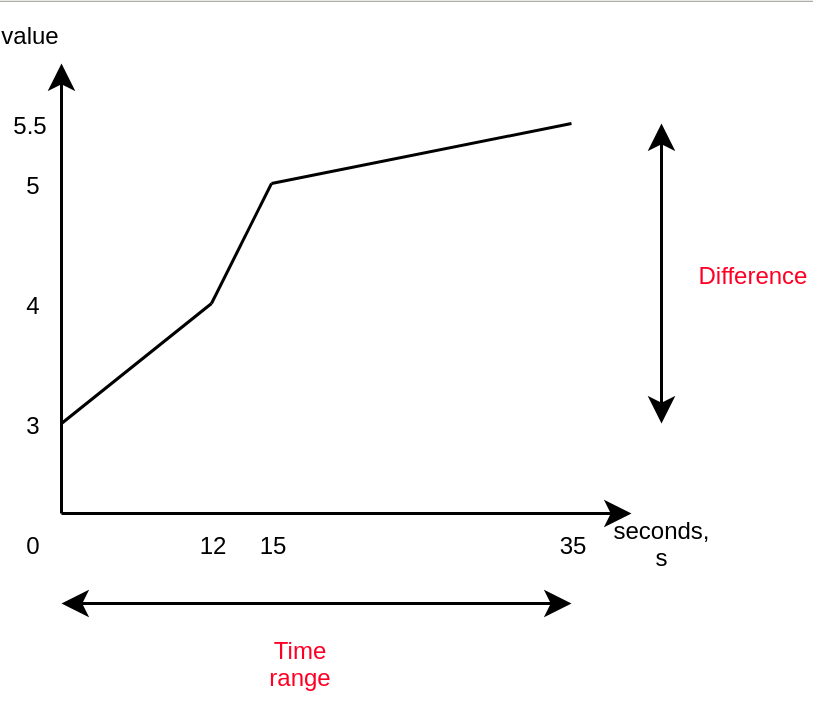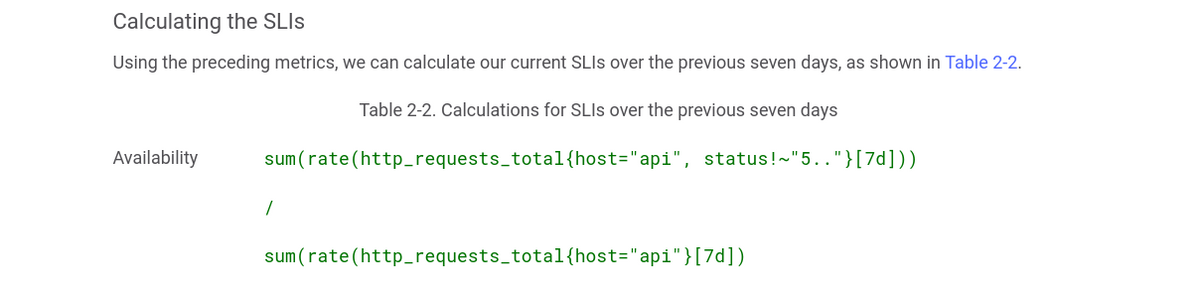# How the Prometheus rate() function works

1. Introduction
2. How It Works
3. Examples

## Introduction

Both Prometheus and its querying language PromQL have quite a few functions for performing various calculations on the data they have. One of the most widely used functions is rate(), however, it is also one of the most misunderstood.

Having a monitoring stack in your company, such as the one that Metricfire provides, gives you the essential functionality that you need; and one of these essential functions is predicting trends. That is where rate() comes into play. As the name suggests, it lets you calculate the per-second average rate of how a value is increasing over a period of time. It is the function to use if you want, for instance, to calculate how the number of requests coming into your server changes over time, or the CPU usage of your servers. But first, let's talk about its internals. We need to understand how it works under the hood so that we can build up our knowledge from there.

If you're interested in trying a Prometheus alternative, you can sign up now for our Hosted Graphite free trial - or sign up for a demo.

‍

## How It Works

### Types of Arguments

There are two types of arguments in PromQL: range and instant vectors. Here is how it would look if we looked at these two types graphically:

‍This is a matrix of three range vectors, where each one encompasses one minute of data that has been scraped every 10 seconds. As you can see, it is a set of data that is defined by a unique set of label pairs. Range vectors also have a time dimension - in this case, it is one minute - whereas instant vectors do not. Here is what instant vectors would look like:

‍‍

As you can see, instant vectors only define the value that has been most recently scraped. rate() and its cousins take an argument of the range type since to calculate any kind of change, you need at least two points of data. They do not return any results at all if there are less than two samples available. PromQL indicates range vectors by writing a time range in square brackets next to a selector which says how much time into the past it should go.

### Choosing the time range for range vectors

What time range should we choose? There is no silver bullet here: at the very minimum, it should be two times the scrape interval. However, in this case, the result will be very “sharp”: all of the changes in the value would reflect in the results of the function faster than any other time range. Thereafter, the result would become 0 again swiftly. Increasing the time range would achieve the opposite - the resulting line (if you plotted the results) would become “smoother” and it would be harder to spot the spikes. Thus, the recommendation is to put the time range into a different variable (let’s say 1m, 5m, 15m, 2h) in Grafana, then you are able to choose whichever value fits your case the best at the time when you are trying to spot something - such as a spike or a trend.

One could also use the special variable in Grafana called \$__interval - it is defined to be equal to the time range divided by the step’s size. It could seem like the perfect solution as it looks like all of the data points between each step would be considered, but it has the same problems as mentioned previously. It is impossible to see both very detailed graphs and broad trends at the same time. Also, your time interval becomes tied to your query step, so if your scrape interval ever changes then you might have problems with very small time ranges.

Something to remember - MetricFire is also a hosted Grafana service. Explore our free trial here - or sign up for a demo.

‍

### Calculation

Just like everything else, the function gets evaluated on each step. But, how does it work?

It roughly calculates the following:

rate(x[35s]) = difference in value over 35 seconds / 35s‍

The nice thing about the rate() function is that it takes into account all of the data points, not just the first one and the last one. There is another function, irate, which uses only the first and last data points.

You might now say… why not delta()? Well, rate() that we have just described has this nice characteristic: it automatically adjusts for resets. What this means is that it is only suitable for metrics that are constantly increasing, a.k.a. the metric type that is called a “counter”. It’s not suitable for a “gauge”. Also, a keen reader would have noticed that using rate() is a hack to work around the limitation that floating-point numbers are used for metrics’ values and that they cannot go up indefinitely so they are “rolled over” once a limit is reached. This logic prevents us from losing old data, so using rate() is a good idea when you need this feature.

Note: because of this automatic adjustment for resets, if you want to use any other aggregation together with rate() then you must apply rate() first, otherwise the counter resets will not be caught and you will get weird results.

Either way, PromQL currently will not prevent you from using rate() with a gauge, so this is a very important thing to realize when choosing which metric should be passed to this function. It is incorrect to use rate() with gauges because the reset detection logic will mistakenly catch the values going down as a “counter reset” and you will get wrong results.

All in all, let’s say you have a counter metric that is changing like this:

• 0
• 4
• 6
• 10
• 2

The reset between “10” and “2” would be caught by irate() and rate() and it would be taken as if the value after that were “12” i.e. it has increased by “2” (from zero). Let’s say that we were trying to calculate the rate with rate() over 60 seconds and we got these 6 samples on ideal timestamps. So the resulting average rate of increase per second would be:

12-0/60 = 0.2. Because everything is perfectly ideal in our situation, the opposite calculation is also true: 0.2 * 60 = 12. However, this opposite calculation is not always true in the cases where some samples are not covering the full range ideally, or when samples do not line up perfectly due to random delays introduced between scrapes. Let me explain this in more detail in the following section.

### Extrapolation: what rate() does when missing information

Last but not least, it’s important to understand that rate() performs extrapolation. Knowing this will save you from headaches in the long term. Sometimes when rate() is executed at a point in time, there might be some data missing if some of the scrapes had failed. What’s more, the scrape interval due to added randomness might not align perfectly with the range vector, even if it is a multiple of the range vector’s time range.

In such a case, rate() calculates the rate with the data that it has and then, if there is any information missing, extrapolates the beginning or the end of the selected window using either the first or last two data points. This means that you might get uneven results even if all of the data points are integers, so this function is suited only for spotting trends, and spikes, and for alerting if something happens.

‍

### Aggregation

Optionally, you apply rate() only to certain dimensions just like with other functions. For example, rate(foo) by (bar) will calculate the rate of change of foo for every bar (label’s name). This can be useful if you have, for example, haproxy running and you want to calculate the rate of change of the number of errors by different backends so you can write something like rate(haproxy_connection_errors_total[5m]) by (backend).

‍

## Examples

Just like described previously, rate() works perfectly in the cases where you want to get an alert when the amount of errors jumps up. So, you could write an alert like this:

‍

``````groups:
- name: Errors
rules:
expr: rate(haproxy_connection_errors_total[5m]) by (backend) > 0.5
for: 10m
labels:
severity: page
annotations:
summary: High connection error count in {{ \$labels.backend }}``````

‍

This would inform you if any of the backends have an increased amount of connection errors. As you can see, rate() is perfect for this use case. Feel free to implement similar alerts for your services that you monitor with MetricFire. Interested to see what we can do for you? Try our free trial or sign up for a demo.

‍

### SLO Calculation

Another common use case for the rate() function is calculating SLIs, and seeing if you do not violate your SLO/SLA. Google has recently released a popular book for site-reliability engineers. Here is how they calculate the availability of the services:

‍‍

As you can see, they calculate the rate of change of the amount of all of the requests that were not 5xx and then divides by the rate of change of the total amount of requests. If there are any 5xx responses then the resulting value would be less than one. You can, again, use this formula in your alerting rules with some kind of specified threshold - then you would get an alert if it is violated or you could predict the near future with predict_linear and avoid any SLA/SLO problems.

Wondering how we can help? MetricFire is a hosted Graphite and Grafana service. We offer a complete infrastructure and application monitoring platform which helps customers collect, store and visualize time series data from any source. If you're interested in trying it out for yourself, sign up for our free trial. You can also sign up for a demo and we can talk about the best monitoring solutions for you.